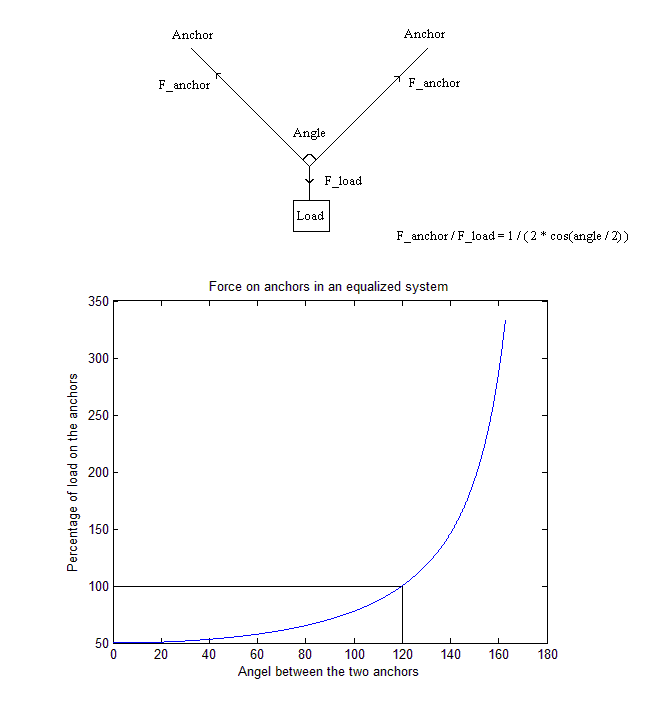## Climbing: Forces on anchors

2014-05-19

A climbing physics lesson!

When I first started climbing I had to find some way to make it geeky, and what followed was this graph:In the diagram on top, the anchors could be bolts, or trees that the ropes are connected to. The load (the climber), is connected to the ropes.

In the graph below, we see what the forces on the anchors depend on the angle between them. If the angle is 0, the load is evenly distributed between the anchors with 50% on each. So ideally, you would want the anchors close together. However, the criteria for this to work is that the system is equalized, that is, both anchors should take an equal amount of weight. That is hard to do when the angle is 0. Somewhere in between 0 and 120 degrees seems to be the best.

It's interesting to note that if the angle is too great, the force on each anchor will actually be larger than 100%. It would actually be less stressful if only one anchor was used.

comments powered by Disqus